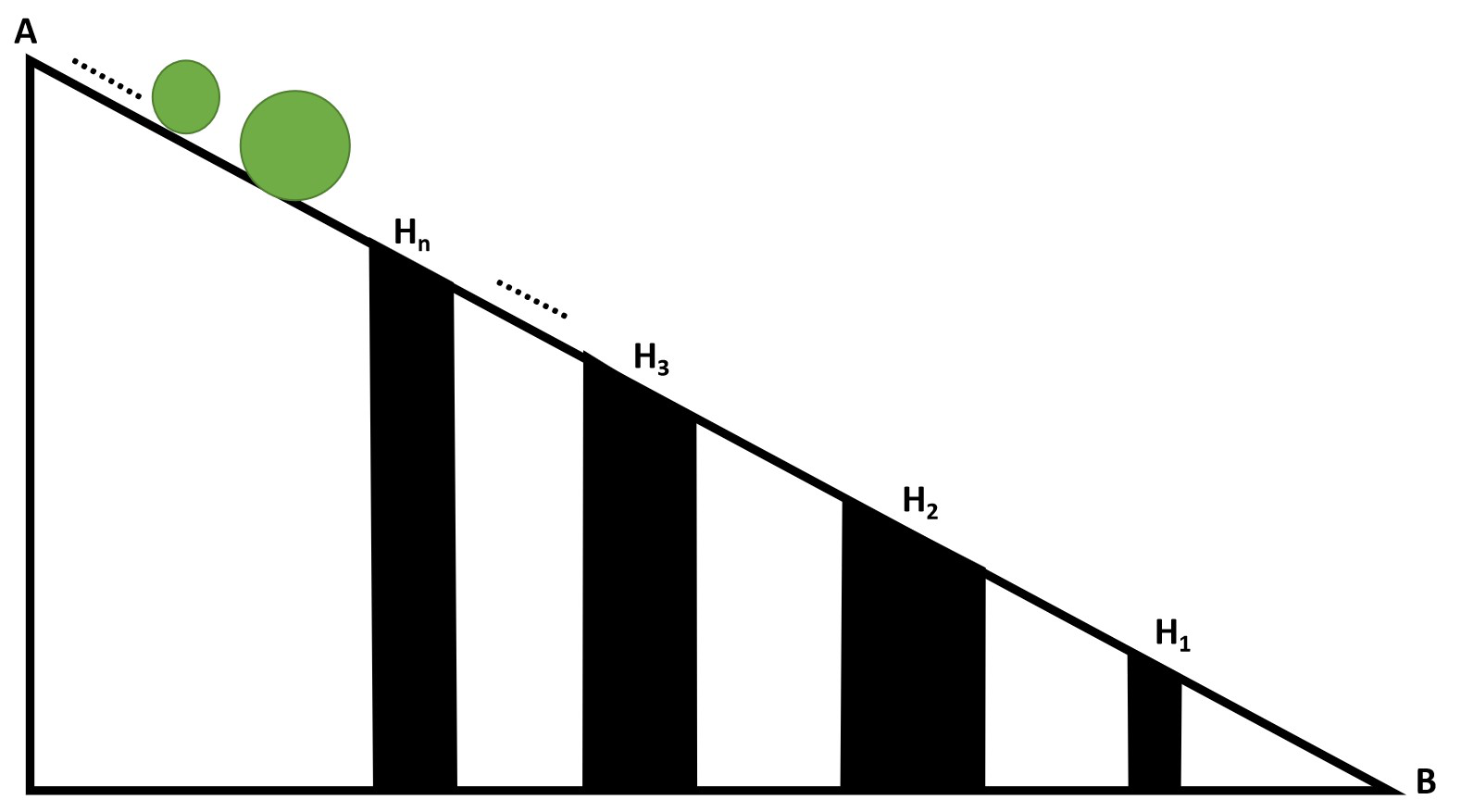Related Articles

# TCS Codevita | Holes And Balls

• Difficulty Level : Medium
• Last Updated : 17 Nov, 2020

Given two arrays of H[] and B[] consisting of N and M integers respectively, denoting the diameter of holes and balls respectively. M number of balls are made to roll from A to B on a sloping surface with N holes, each having different depth as shown in the figure below:The task is to find the eventual position of each ball in the order of the ball released considering the following:

• A ball will fall into a hole if its diameter is less than or equal to the diameter of the hole.
• A hole Hi will become full if i numbers of balls fall into it.
• If a hole is full, then no more balls fall into it.
• A ball will reach B from A, if and only if it is not falling into any one of the holes.
• If a ball is in hole Pi, then its position is i. If a ball reached the bottom point B, then take its position as 0.

Examples:

Input: H[] = {21, 3, 6}, B[] = {20, 15, 5, 7, 10, 4, 2, 1, 3, 6, 8}
Output: 1 0 3 0 0 3 3 2 2 0 0
Explanation:
Ball of diameter 20 will fall into the hole H1 and the hole H1 will become full.
Balls with diameter 15, 7 and 10 will reach bottom, since the hole H1 is full and diameters of holes H2 and H3 are less than the diameters of the balls.
Balls with diameters 5, 4 and 2 will fall into the hole H3.
Ball with diameter 1 will fall into the hole H2 since the hole H3 is already full.
Ball with diameter 3 will fall into hole H2.
Balls with diameters 6, and 8 will reach the bottom point B.
The position of ball 20 is 1 because it is in hole H1.
Positions of ball 15, 7, 10, 3, 6, and 8 are 0 because they reached the bottom point B.
Therefore, the balls with diameter 5, 4 and 2 are in the 3rd hole H3, the ball with diameter 1 and 3 are in the 2nd hole H2.

Input: H[] = {20, 15, 10, 5, 25}, B[] = {5, 10, 15, 20, 25, 30, 4, 9, 14, 19}
Output: 5 5 5 5 5 0 4 3 2 1

## Recommended: Please try your approach on {IDE} first, before moving on to the solution.

Approach: Follow the steps below to solve the problem:

• Initialize an array position[] of size N to store the final position of each ball and an array depth[] of size N to store the capacity of each hole.
• Iterate over the range [1,  N] using the variable i and set the initial depth[i] of the hole[i] to i+1.
• Traverse the array ball[] using the variable i and do the following:
• Iterate over the array hole[] using variable j in reverse order.
• Check if the diameter of the hole is greater than or equal to that of the ball, i.e., hole[j] ≥ ball[i], and if that hole is not full, i.e., depth[j] > 0 then, place the ball in that hole by appending j + 1 in the position[] array and decrementing the depth of the hole by 1 and break out of the loop.
• If the ball doesn’t fit in any hole (has reached at end of the slope), then append 0 in the position[] array.
• After the above steps, print the value stored in the array position[] as the result.

Below is the implementation of the above approach:

## Python3

 `# Python program for the above approach`` ` `# Function to find and print the final``# position of balls``def` `ballPositionFinder(diameter_of_holes,``                       ``diameter_of_balls):``   ` `    ``max_hole_limit_counter ``=` `[]``    ``position_value ``=` `[]``     ` `    ``# Stores the positions of balls``    ``ball_positions ``=` `[]`` ` `    ``# Determine the maximum balls a hole``    ``# can store and note the position``    ``# of holes in position_value``    ``for` `i ``in` `range``(``1``, ``len``(diameter_of_holes)``+``1``):``        ``max_hole_limit_counter.append(i)``        ``position_value.append(i)`` ` `    ``# Iterate over all possible holes``    ``# for every ball released``    ``for` `i ``in` `range``(``0``, ``len``(diameter_of_balls)):``        ``for` `j ``in` `range``(``1``, ``len``(diameter_of_holes)``+``1``):`` ` `            ``# Place ball in hole if it fits``            ``# in and if hole is not full``            ``if` `(diameter_of_holes[``-``j] >``=` `diameter_of_balls[i]) ``and` `(``                    ``max_hole_limit_counter[``-``j] !``=` `0``):``               ` `                ``ball_positions.append(position_value[``-``j])``                ``max_hole_limit_counter[``-``j] ``-``=` `1``                ``break`` ` `            ``# If ball has reached at end B``            ``if` `j ``=``=` `len``(diameter_of_holes):``                ``ball_positions.append(``0``)``                ``break`` ` `    ``return` `ball_positions`` ` ` ` `# Driver Code``if` `__name__ ``=``=` `"__main__"``:``   ` `    ``diameter_of_holes ``=` `[``21``, ``3``, ``6``]``     ` `    ``diameter_of_balls ``=` `[``20``, ``15``, ``5``, ``7``, ``10``, ``4``,``                         ``2``, ``1``, ``3``, ``6``, ``8``]`` ` `    ``# Function Call``    ``output ``=` `ballPositionFinder(diameter_of_holes,``                                ``diameter_of_balls)``    ``print``(``*``output, sep ``=``' '``)`
Output:
```1 0 3 0 0 3 3 2 2 0 0
```

Time Complexity: O(N*M)
Auxiliary Space: O(N)

Attention reader! Don’t stop learning now. Get hold of all the important DSA concepts with the DSA Self Paced Course at a student-friendly price and become industry ready.  To complete your preparation from learning a language to DS Algo and many more,  please refer Complete Interview Preparation Course.

In case you wish to attend live classes with experts, please refer DSA Live Classes for Working Professionals and Competitive Programming Live for Students.

My Personal Notes arrow_drop_up